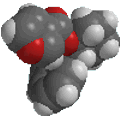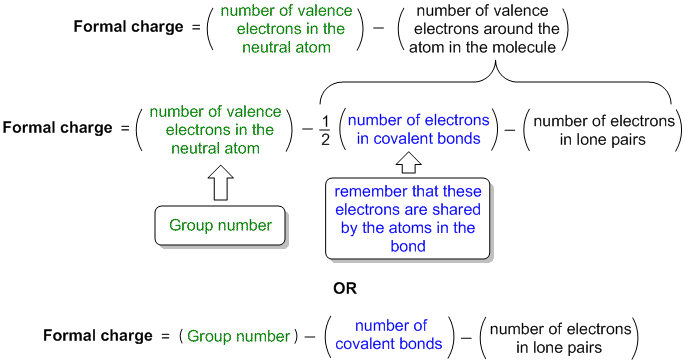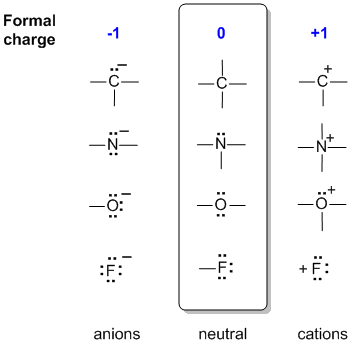Chapter 1: Structure Determines PropertiesFormal Charges
• Not all atoms within a neutral molecule need be neutral.
• The location of any charges is often useful for understanding or predicting reactivity.
• Identifying formal charges helps you keep track of the electrons.
• It's a good idea to get into the habit of automatically labelling the formal charges on any atoms within a molecule.
• The formal charge on an atom can be calculated using a mathematical equation, a diagram or by instinct (!)
• For organic molecules in general, the majority of atoms will usually be neutral and the most common charges are +/- 1 (except on metals).
• Check out some questions ?

Knowing the formal charge on a particular atom in a structure is an important part of keeping track of the electrons and is important for establishing and predicting the reactivity.
The formal charge on an atom in a molecule reflects the electron count associated with the atom compared to the isolated neutral atom. If the atom has given away electrons it will be +ve and if it has gained electrons it will be -ve.

Although formal charge can be calculated via a mathematical formula, or a diagram, it is also possible to do it "instinctively" based on comparing structures.  The "instinctive method" is quicker, and is probably what your instructor uses, but it does require more skill and an experience of common structures.

Formal charge equation formally compares the number of valence electrons in an isolated neutral atom (which can be determined from the older style group number of the periodic table) with the number of valence electons around the atom in the molecule:Diagramatic or visual method visually compares the number of valence electrons in an isolated neutral atom (which can be determined from the older style group number of the periodic table) with the atom in the molecule. It's a visual equivalent of the equation based mthod described above.

• Draw a circle around the atom of interest
• Count the number of electrons in the atoms circle (electrons in covalent bonds are shared)
• Formal charge = group number of atom of interest - electrons in the circle of atom of interestExample molecule of interestFormal charge on oxygen: Group number = 6 Number of covalent bonds = 2 Number of lone pair electrons = 4Formal charges for all the different atoms

Instinctive method
This is based on comparing the structure with common, known neutral structures.  To do this you need to recognise the common neutral structures: C 4 bonds, N 3 bonds, 1 lone pair, O 2 bonds, 2 lone pairs, F 1 bond, 3 lone pairs.  Notice how these numbers work in relation to the octet rule (4 pairs). Once mastered, this is much quicker.

In the middle of the following diagram are the neutral bonding situations for C, N, O and a halogen, F. (For example, just think of each central atom as being bonded to hydrogen atoms).

To the left, a bond has been lost but converted to a lone pair, so the central atom has gained an electron and become -ve.

To the right, there are 2 scenarios:

• C and F have lost the shared electrons of a bond, so losing one electron from the "count" to become +ve.
• N and O have converted two unshared electrons into a shared pair of electrons in a bond, so losing one electron from the "count" to become +veNow would be a good time to check out some questions© Dr. Ian Hunt, Department of Chemistry, University of Calgary